Documentation

## Structure From Motion From Two Views

Structure from motion (SfM) is the process of estimating the 3-D structure of a scene from a set of 2-D images. This example shows you how to estimate the poses of a calibrated camera from two images, reconstruct the 3-D structure of the scene up to an unknown scale factor, and then recover the actual scale factor by detecting an object of a known size.

### Overview

This example shows how to reconstruct a 3-D scene from a pair of 2-D images taken with a camera calibrated using the Camera Calibrator app. The algorithm consists of the following steps:

1. Match a sparse set of points between the two images. There are multiple ways of finding point correspondences between two images. This example detects corners in the first image using the `detectMinEigenFeatures` function, and tracks them into the second image using `vision.PointTracker`. Alternatively you can use `extractFeatures` followed by `matchFeatures`.

2. Estimate the fundamental matrix using `estimateFundamentalMatrix`.

3. Compute the motion of the camera using the `relativeCameraPose` function.

4. Match a dense set of points between the two images. Re-detect the point using `detectMinEigenFeatures` with a reduced `'MinQuality'` to get more points. Then track the dense points into the second image using `vision.PointTracker`.

5. Determine the 3-D locations of the matched points using `triangulate`.

6. Detect an object of a known size. In this scene there is a globe, whose radius is known to be 10cm. Use `pcfitsphere` to find the globe in the point cloud.

7. Recover the actual scale, resulting in a metric reconstruction.

### Read a Pair of Images

Load a pair of images into the workspace.

```imageDir = fullfile(toolboxdir('vision'), 'visiondata','upToScaleReconstructionImages'); images = imageDatastore(imageDir); I1 = readimage(images, 1); I2 = readimage(images, 2); figure imshowpair(I1, I2, 'montage'); title('Original Images'); ```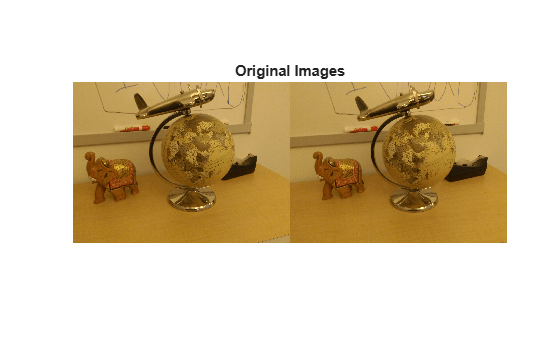This example uses the camera parameters calculated by the cameraCalibrator app. The parameters are stored in the `cameraParams` object, and include the camera intrinsics and lens distortion coefficients.

```% Load precomputed camera parameters load upToScaleReconstructionCameraParameters.mat ```

### Remove Lens Distortion

Lens distortion can affect the accuracy of the final reconstruction. You can remove the distortion from each of the images using the `undistortImage` function. This process straightens the lines that are bent by the radial distortion of the lens.

```I1 = undistortImage(I1, cameraParams); I2 = undistortImage(I2, cameraParams); figure imshowpair(I1, I2, 'montage'); title('Undistorted Images'); ```### Find Point Correspondences Between The Images

Detect good features to track. Reduce `'MinQuality'` to detect fewer points, which would be more uniformly distributed throughout the image. If the motion of the camera is not very large, then tracking using the KLT algorithm is a good way to establish point correspondences.

```% Detect feature points imagePoints1 = detectMinEigenFeatures(rgb2gray(I1), 'MinQuality', 0.1); % Visualize detected points figure imshow(I1, 'InitialMagnification', 50); title('150 Strongest Corners from the First Image'); hold on plot(selectStrongest(imagePoints1, 150)); % Create the point tracker tracker = vision.PointTracker('MaxBidirectionalError', 1, 'NumPyramidLevels', 5); % Initialize the point tracker imagePoints1 = imagePoints1.Location; initialize(tracker, imagePoints1, I1); % Track the points [imagePoints2, validIdx] = step(tracker, I2); matchedPoints1 = imagePoints1(validIdx, :); matchedPoints2 = imagePoints2(validIdx, :); % Visualize correspondences figure showMatchedFeatures(I1, I2, matchedPoints1, matchedPoints2); title('Tracked Features'); ```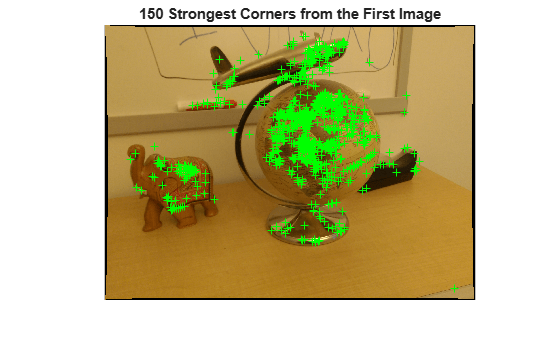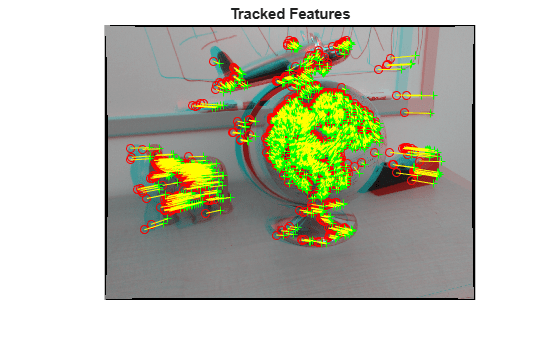### Estimate the Essential Matrix

Use the `estimateEssentialMatrix` function to compute the essential matrix and find the inlier points that meet the epipolar constraint.

```% Estimate the fundamental matrix [E, epipolarInliers] = estimateEssentialMatrix(... matchedPoints1, matchedPoints2, cameraParams, 'Confidence', 99.99); % Find epipolar inliers inlierPoints1 = matchedPoints1(epipolarInliers, :); inlierPoints2 = matchedPoints2(epipolarInliers, :); % Display inlier matches figure showMatchedFeatures(I1, I2, inlierPoints1, inlierPoints2); title('Epipolar Inliers'); ```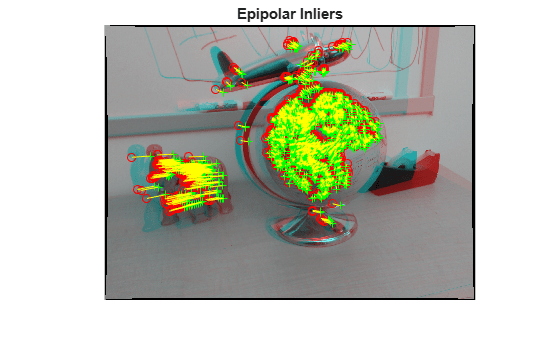### Compute the Camera Pose

Compute the location and orientation of the second camera relative to the first one. Note that `t` is a unit vector, because translation can only be computed up to scale.

```[orient, loc] = relativeCameraPose(E, cameraParams, inlierPoints1, inlierPoints2); ```

### Reconstruct the 3-D Locations of Matched Points

Re-detect points in the first image using lower `'MinQuality'` to get more points. Track the new points into the second image. Estimate the 3-D locations corresponding to the matched points using the `triangulate` function, which implements the Direct Linear Transformation (DLT) algorithm . Place the origin at the optical center of the camera corresponding to the first image.

```% Detect dense feature points. Use an ROI to exclude points close to the % image edges. roi = [30, 30, size(I1, 2) - 30, size(I1, 1) - 30]; imagePoints1 = detectMinEigenFeatures(rgb2gray(I1), 'ROI', roi, ... 'MinQuality', 0.001); % Create the point tracker tracker = vision.PointTracker('MaxBidirectionalError', 1, 'NumPyramidLevels', 5); % Initialize the point tracker imagePoints1 = imagePoints1.Location; initialize(tracker, imagePoints1, I1); % Track the points [imagePoints2, validIdx] = step(tracker, I2); matchedPoints1 = imagePoints1(validIdx, :); matchedPoints2 = imagePoints2(validIdx, :); % Compute the camera matrices for each position of the camera % The first camera is at the origin looking along the Z-axis. Thus, its % rotation matrix is identity, and its translation vector is 0. camMatrix1 = cameraMatrix(cameraParams, eye(3), [0 0 0]); % Compute extrinsics of the second camera [R, t] = cameraPoseToExtrinsics(orient, loc); camMatrix2 = cameraMatrix(cameraParams, R, t); % Compute the 3-D points points3D = triangulate(matchedPoints1, matchedPoints2, camMatrix1, camMatrix2); % Get the color of each reconstructed point numPixels = size(I1, 1) * size(I1, 2); allColors = reshape(I1, [numPixels, 3]); colorIdx = sub2ind([size(I1, 1), size(I1, 2)], round(matchedPoints1(:,2)), ... round(matchedPoints1(:, 1))); color = allColors(colorIdx, :); % Create the point cloud ptCloud = pointCloud(points3D, 'Color', color); ```

### Display the 3-D Point Cloud

Use the `plotCamera` function to visualize the locations and orientations of the camera, and the `pcshow` function to visualize the point cloud.

```% Visualize the camera locations and orientations cameraSize = 0.3; figure plotCamera('Size', cameraSize, 'Color', 'r', 'Label', '1', 'Opacity', 0); hold on grid on plotCamera('Location', loc, 'Orientation', orient, 'Size', cameraSize, ... 'Color', 'b', 'Label', '2', 'Opacity', 0); % Visualize the point cloud pcshow(ptCloud, 'VerticalAxis', 'y', 'VerticalAxisDir', 'down', ... 'MarkerSize', 45); % Rotate and zoom the plot camorbit(0, -30); camzoom(1.5); % Label the axes xlabel('x-axis'); ylabel('y-axis'); zlabel('z-axis') title('Up to Scale Reconstruction of the Scene'); ```### Fit a Sphere to the Point Cloud to Find the Globe

Find the globe in the point cloud by fitting a sphere to the 3-D points using the `pcfitsphere` function.

```% Detect the globe globe = pcfitsphere(ptCloud, 0.1); % Display the surface of the globe plot(globe); title('Estimated Location and Size of the Globe'); hold off ```### Metric Reconstruction of the Scene

The actual radius of the globe is 10cm. You can now determine the coordinates of the 3-D points in centimeters.

```% Determine the scale factor scaleFactor = 10 / globe.Radius; % Scale the point cloud ptCloud = pointCloud(points3D * scaleFactor, 'Color', color); loc = loc * scaleFactor; % Visualize the point cloud in centimeters cameraSize = 2; figure plotCamera('Size', cameraSize, 'Color', 'r', 'Label', '1', 'Opacity', 0); hold on grid on plotCamera('Location', loc, 'Orientation', orient, 'Size', cameraSize, ... 'Color', 'b', 'Label', '2', 'Opacity', 0); % Visualize the point cloud pcshow(ptCloud, 'VerticalAxis', 'y', 'VerticalAxisDir', 'down', ... 'MarkerSize', 45); camorbit(0, -30); camzoom(1.5); % Label the axes xlabel('x-axis (cm)'); ylabel('y-axis (cm)'); zlabel('z-axis (cm)') title('Metric Reconstruction of the Scene'); ```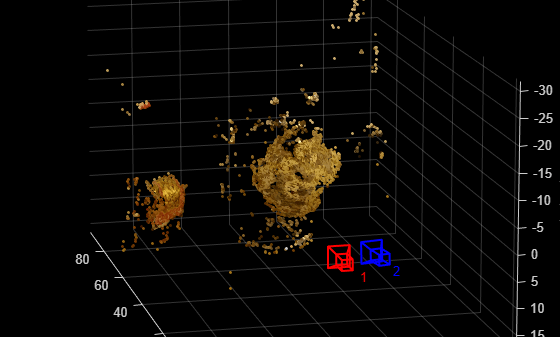### Summary

This example showed how to recover camera motion and reconstruct the 3-D structure of a scene from two images taken with a calibrated camera.

### References

 Hartley, Richard, and Andrew Zisserman. Multiple View Geometry in Computer Vision. Second Edition. Cambridge, 2000.